# Line segment

Cut a line segment of 15 cm into two line segments so that their lengths are in ratio 2:1. What length will each have?

Result

a =  10 cm
b =  5 cm

#### Solution:

a+b = 15
a = 2b

a+b = 15
a-2b = 0

a = 10
b = 5

Calculated by our linear equations calculator.

Leave us a comment of example and its solution (i.e. if it is still somewhat unclear...):

Showing 0 comments:Be the first to comment!#### To solve this example are needed these knowledge from mathematics:

Do you have a linear equation or system of equations and looking for its solution? Or do you have quadratic equation?

## Next similar examples:

1. Line segmentThe 4 cm long line segment is enlarged in the ratio of 5/2. How many centimeters will measure the new line segment?
2. Find midpoint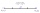FM=5y+13, MG=5-3y, FG=? M is the midpoint of FG. Use the given information to find the missing measure or value.
3. Louvers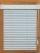The company charges for office equipment louvers total 262 Eur. From the delivery bill is obvious that the louvers are 76 Eur expensive than their installation. What percentage of the total charged is the installation of louvers?
4. Number ratio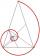Calculate two positive numbers that its ratio is 6:6 and difference was 0.
5. Unknown numberIdentify unknown number which 1/5 is 40 greater than one tenth of that number.
6. Two numbers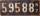Determine the numbers x and y so x + y = 8 is truth and the numbers are in the ratio of 4: 5.
7. AgesJohn, Teresa, Daniel and Paul have summary 56 years. Their ages are in a ratio of 1:2:5:6. Determine how many years have each of them.
8. PeppersIn the box are yellow (a), green (b) and red (c) peppers. Their amount is in a ratio 2:4:1 . Most are yellow peppers and green the least. Calculate the number of peppers each type if the total number of peppers is 70.
9. Land areaA land area of Asia and Africa are in a 3: 2 ratio, the European and African are is 1:3. What are the proportions of Asia, Africa, and Europe?
10. Image scale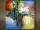The actual image dimensions are 60 cm x 80cm and has a reduced size 3 cm x 4 cm. At what scale the image was reduced?
11. Unknown number 24f we add 20, we get 50% of its triple. What is this unknown number?
12. 1.5 divided1.5 divided by 1 = w divided by 4
13. DonutsFind how many donuts each student will receive if you share 126 donuts in a ratio of 1:5:8
14. Divide 5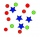Divide 288 in the following ratio 3 : 4 : 5
15. The farmer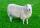The farmer had 140 sheep. For the next year, she decided to change the number of sheep in ratio 10: 7. How many sheep will he have then?
16. Simple equationSolve for x: 3(x + 2) = x - 18
17. EquationSolve the equation: 1/2-2/8 = 1/10; Write the result as a decimal number.